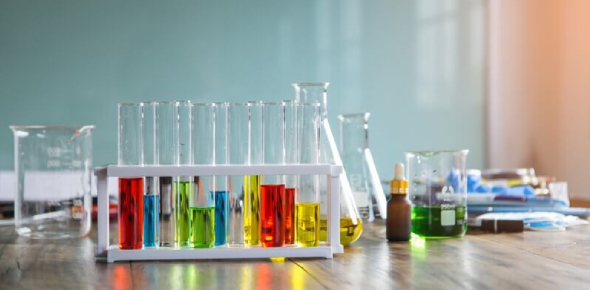# Chemistry 103 Quiz: Trivia Questions!

15 Questions | Total Attempts: 175SettingsWhy should we care about chemistry? If you’re asking yourself this question, you do not realize how important chemistry is to our daily lives. Chemistry is imperative for our basic needs, such as food, clothing, shelter, health, energy, clean water, and soil. Chemicals also help us to find new cures for deadly diseases or treatments for illnesses. Science is the reason why we now have a COVID vaccine. This quiz will show you how valuable chemistry is.

• 1.
Write the formula between ionic compounds K and S?
• A.

KS2

• B.

K3S2

• C.

K2S

• D.

K2S3

• 2.
What is the molar mass Ba(OH)2 .8H2O?
• A.

281.4 g/mol

• B.

298.4 g/mol

• C.

299.5 g/mol

• D.

315.5 g/mol

• 3.
Convert 5 km/h  to  m/sec?
• A.

1.5 m/sec

• B.

1.37 m/sec

• C.

1.39 m/sec

• D.

2 m/sec

• 4.
When 5g of H2 and 5g of O2 were reacted, the yield of H2O was 87.9%. What is the actual yield of H2O? 2H2 + O2 ——> 2H2O
• A.

6.4 g

• B.

4.9 g

• C.

5.1 g

• D.

12.8 g

• 5.
Calculate the mass in gram of cube if density = 19.32 g/cm3 and the length of the cube is 2 cm.
• A.

154.56 g

• B.

155 g

• C.

154.1 g

• D.

156 g

• 6.
Which one of the following is a correct expression for molarity?
• A.

Mol solute / L solvent

• B.

Mol solute / ml solvent

• C.

Mmol solute / ml solution

• D.

Mmol solute / L solution

• 7.
The group 6A called?
• A.

Halogens

• B.

Noble gases

• C.

Alkali metal

• D.

Chalcogens

• 8.
50.0 ml of water were added to 150 ml of 5.0 M H2SO4 solution, calculate the concentration of the solution.
• A.

3.3 M

• B.

3.5 M

• C.

4.00 M

• D.

3.75 M

• 9.
Round 0.00758 to two significant figures.
• A.

0.0080

• B.

0.00750

• C.

0.0076

• D.

0.0075

• 10.
How many Oxygen atoms are in 0.88 mol of C6H12O6? ( N.A = 6.02 *10^23 )
• A.

31*10^23

• B.

31.8*10^23

• C.

32*10^24

• D.

5.3*10^23

• 11.
What is the concentration M of Na+ in a solution by dissolving 23.4 g of Na2CO3 in 125.0 ml of solution? M.w(Na2CO3) = 106 g/mol
• A.

3.4 M

• B.

3.53 M

• C.

3.00 M

• D.

4.1 M

• 12.
The charge of element Ra?
• A.

+1

• B.

-1

• C.

+2

• D.

Non of the above

• 13.
6.5 grams of Al reacts with excess of Oxygen. How many grams of Al2O3 is formed ? ( M.W Al = 27 g/mol . M.W Al2O3 = 102 g/mol )  4Al + 3O2 ——> 2Al2O3
• A.

11.2 g

• B.

12.1 g

• C.

12.24 g

• D.

13.35 g

• 14.
Calculate the molarity of NaOH solution if 10.42 ml of this solution are needed to neutralize 25.00 ml of 0.2043 M oxalic acid ( H2C2O4) ?
• A.

1.500 M

• B.

0.9803 M

• C.

0.4902 M

• D.

0.2500 M

• 15.
Carry out the following operation  ( 4.02+5.612 ) ( 15.2-15.15 ) =
• A.

0.48

• B.

0.4

• C.

0.5

• D.

0.4816

Related TopicsBack to top